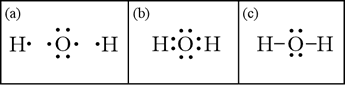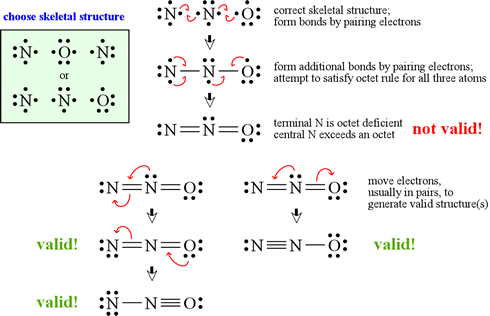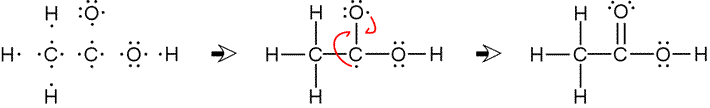CHEM 101
General Chemistry

J. D. Cronk    Syllabus    Topics

GENERAL CHEMISTRY TOPICS

### Lewis structures

Examples of how to draw Lewis structures: Water (H2O), Dinitrogen monoxide (Nitrous oxide, N2O), acetic acid (C2H4O2). General rules for drawing Lewis structures.

Lewis structures are structural formulas for molecules and polyatomic ions that represent all valence electrons. Since valence electrons are typically represented as dots, these structural formulas sometimes are called Lewis dot stutctures. Here we present some examples of how to draw Lewis structures. The general rules for drawing Lewis structures are given below.

Example 1. Water. The formula for water is H2O. Counting valence electrons yields eight total (six from oxygen, one each from the two hydrogens). Hydrogen is special since it can only accommodate a duet, and therefore can form at most one bond. Atoms that can only form one bond must be terminal (or peripheral) atoms in the structure.The skeletal structure for water must be H O H, and not H H O, even though hydrogen has lower electronegativity than oxygen (compare this case to that of N2O, which follows a general rule that less electronegative atoms tend to be central atoms in Lewis structures).

In panel (a), the skeletal structure for water is shown with the atoms represented by their Lewis symbols. The eight total valence electrons are explicitly represented. In (b) we allow single electrons - one each from hydrogen and oxygen - to form a bonding pair between the nuclei. In (c), we have replaced both bonding pairs with a line (or dash) to symbolize the covalent bond formed between the atoms by the bonding electron pair. Note that oxygen is surrounded by an octet of electrons, satisfying the octet rule. We have drawn a valid Lewis structure for water.

Example 2. Dinitrogen monoxide (Nitrous oxide, N2O)
. Since either N or O can serve as a central atom, in choosing a skeletal structure we use the rule that the least electronegative atom be placed in the middle.Having the appropriate skeletal structure and correct count of valence electrons, the goal is to place the electrons in bonds or lone pairs so that each atom has an octet of electrons. The figure at left shows a procedure for generating candidate structures by moving electrons singly, or in pairs, starting with the Lewis symbols for the elements. Since N and O are both Period 2 elements, no atom can exceed an octet. In this case, it is possible to draw three valid Lewis structures.

These three valid Lewis structures for dinitrogen monoxide are known as non-equivalent resonance structures. As an assessment tool, formal charge assignments can be used to predict the relative contributions of the resonance forms to the resonance hybrid, which represents a more realistic conception of the electron distribution within the molecule.

Example 3. Acetic acid (C2H4O2). There are cases where even for very simple molecules there are several chemically plausible skeletal structures. For structures of larger molecules, in which more than one atom is joined to two or more atoms, there is no longer a unique central atom, and the probability of encountering isomers dramatically increases. In order to draw the Lewis structure for a given isomer, more information about the skeletal structure is necessary. Such is the case for most organic molecules, those containing primarily carbon, hydrogen, and oxygen, with other elements such as nitrogen, sulfur, and phosphorous also common in molecules of biological importance. An example is acetic acid, an important example of a weak acid. For acetic acid, the skeletal structure is centered on a chain of atoms bonded together as C C O. The first carbon atom uses its three remaining valence electrons to form bonds to three of the four hydrogens. To the second carbon atom is attached another oxygen atom. Finally, the remaining hydrogen atom attaches to one of the oxygen atoms.In this example, once the skeletal structure is specified, a Lewis structure follows fairly readily. Drawing bonds in place of pairs of electrons composed of one electron from each atom yields a bonded skeletal structure. The octet rule can be satisfied for all non-hydrogen atoms when the remaining unpaired electrons are moved in to form a double bond between carbon and oxygen.

#### Rules for drawing Lewis structures

Goal: Given a chemical formula corresponding to a molecule or molecular ion, draw a Lewis structure.

1. First of all, a correct count of all valence electrons is essential. One way to do this is to write the Lewis symbols for all of the atoms in the formula, and count up all the "dots". For a molecule (uncharged), that count is the correct number of valence electrons. For polyatomic ions, total the valence electrons for all atoms in the formula and subtract one electron for each positive charge of a cation, and add one electron for each unit negative charge of an anion.

2. Draw a skeletal structure. What this means is that we decide how the atoms are to be bonded. Choose a central atom (we'll start with small molecule examples for which there is only one central atom, and the other atoms - the peripheral atoms - are all bonded to the central atom). Hydrogen (H) and fluorine (F) each have valence of 1, and generally these will not be central atoms (bonded to more than one atom). Given a formula, the central atom is typically the first atom (ClF4 example), although this convention is not always followed (e.g. HNO3). Another good way to choose is to pick the least electronegative atom. Inevitably, there will be cases where it is possible to draw more than one skeletal structure.

3. Draw bonds as lines between atoms. Each bond counts as 2 e.

4. Add electrons as non-bonding lone pairs around the peripheral atoms so that they have octets (eight electrons total). Note that this does not apply to H, which can only accommodate a duet (2 e).

5. Add remaining pair(s) of electrons to the central atom so that its octet is complete (if not already). Never exceed an octet for a period 2 atom! For periods 3 and greater, atoms are large enough to accommodate more than an octet in their valence bonding shell. If there are no further electrons available and the central still does not have a complete octet, a lone pair on a peripheral atom may be pushed into a second (or third) bond with the central atom. Carbon and nitrogen are 2nd period elements that commonly form double and triple bonds as central atoms, and oxygen as a peripheral atom is often found in a double bond with the central atom.

6. If all atoms from the 2nd period and greater have at least an octet, and no 2nd period atom exceeds an octet, and the total number of electrons in bonds and lone pairs is equal to the total number of valence electrons available, then a valid Lewis structure has been produced. The convention for ions is to enclose the structure in brackets, and indicate the net charge at the upper right corner.

7. Evaluation, exceptions, and use. Note that there are a few cases where the best Lewis structure has an incomplete octet on a central atom. Since it is often possible to draw more than one valid Lewis structure for a molecule or molecular ion, we will need evaluate which one(s) are more plausible or make better chemical sense. As noted above, formal charge is used as a guide in that total number of formal charges zero or a minimum is generally best, and the formal charge of an atom is considered in relation to its electronegativity. Remember, a Lewis structure is not the molecule, but only a shorthand symbolism that is meant to convey some information about it. Such information informs prediction of the likely physical or chemical properties of the real molecule or the bulk substance that is made up of those molecules. One of the most common uses we make of a valid Lewis structure (and for this we do not need the best Lewis structure, just any valid one) is to predict molecular shape and polarity.

#### Octet rule

Whenever possible, the valence electrons are distributed in such a way that eight electrons (an octet of electrons) surround each main-group element (except hydrogen, which should have two electrons).

Tip: Never exceed an octet for a period 2 atom.